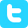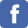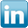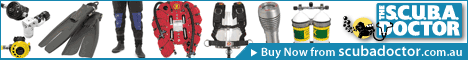search
> contact Lloyd
> Lloyd's blog
> forums# Surface Air Consumption (SAC) Rate Calculator

Disclaimer: I take no responsibility for the accuracy of the calculations and data below. Always verify against other sources.

This calculator can be used to find the Surface Air Consumption (SAC) in litres per minute based on the provided average diving depth in metres, length of time at that depth in minutes, how may bar of breathing gas was used, and the size of the cylinder used in litres. Just enter values for "Time at Depth", "Average Depth", "Gas Used", and "Cylinder Size" and click on the "Submit" button.

SAC Calculator
* Indicates required field
 *Time at Depth e.g. 30 minutes duration time *Average Depth e.g. 20 metres *Gas Used e.g. 150 bar of cylinder pressure *Cylinder Size e.g. 12.2 litres of cylinder water capacity

Diving for 30 minutes at an Average Depth of 20 metres with Gas Used of 150 bar from a Cylinder Size of 12.2 litres
your Surface Air Consumption (SAC) rate rounding up is:

## 20.4 litres per minute

Disclaimer:
This calculator provides information about gas use in scuba diving. UNDER NO CIRCUMSTANCES is there any implication that any gas mixture is safe or even life supporting at any depth. If you have not recived training in scuba diving, you should not attempt to use any of these results. Improper use of gasses in diving can result in injury or DEATH.

## Explanation

When planning technical dives it's essential to calculate if there is sufficient breathing gas to complete the dive. Gas management planning strategies vary, and there are many combinations and permutations of dive scenarios that determine exactly which strategy will be adopted.

In order to calculate the quantity of breathing gas required, we need to be able to estimate our gas consumption rate under various diving conditions. Typically divers will work out their consumption rates for during the descent phase, working on the bottom, the ascent phase, and resting at decompression and/or safety stops.

This is done by recording the Average Depth in metres for the part of the dive under scrutiny, the cylinder pressure in bar at the start of the period, the cylinder pressure in bar at the end of the period, the Cylinder Size in litres based on its water capacity, and the Time at Depth for the duration of the part of the dive. By subtracting the starting cylinder pressure from the end cylinder pressure we get the Gas Used in bar for this part of the dive.

From this information we can calulate the breathing gas consumption rate at depth and convert it to an equivalent consumption rate at the surface - surface air consumption (SAC) rate - which we can then use in planning subsequent dives at various depths.

## Formula

To calculate the equivalent air depth (EAD) using Nitrox for a specific FO2 and planned diving depth, the following formulas are used:

#### Metric - metres

SAC (lpm) = ((Gas Used * Cylinder Size) / ((Average Depth / 10) + 1)) / Time at Depth

In which Gas Used in bar is the cylinder pressure at the start of the period, minus the cylinder pressure at the end of the period, Cylinder Size is the water capacity of the cylinder in litres, Average Depth is the average depth for the period of the dive in metres, Time at Depth is the duration of the period of the dive in mintues, and SAC is the surface air consumption rate in litres per minute. For example, if we use 150 bar of gas from a cylinder with a water capacity size of 12.2 litres, at an average depth of 20 metres for a time at depth of 30 minutes,
the SAC (lpm) is (((150 * 12.2) / ((20 / 10) + 1)) / 30) = 20.4 litres per minute.

[ previous | up ]